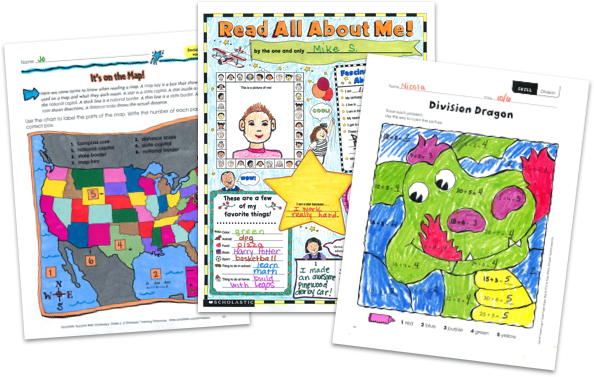MY FILE CABINET# Math Printables From Scholastic TeachablesScholastic Teachables (formerly Scholastic Printables) has more than 6,000 math printables for grades Pre-K through 8 that span a wide range different math skills! Sign up today and get instant online access to math worksheets, math lesson plans, practice pages, activities, games, puzzles, test prep, and more to cover all grades and skills.

Scholastic Teachables (formerly Scholastic Printables) has more than 6,000 math printables for grades Pre-K through 8 that span a wide range different math skills! Sign up today and get instant online access to math worksheets, math lesson plans, practice pages, activities, games, puzzles, test prep, and more to cover all grades and skills.

## Scholastic's Math Printables Cover:

• Charts and Graphs
• Data Analysis
• Decimals
• Early Math
• Estimation
• Fractions
• Geometry
• Glyphs
• Logic and Problem Solving
• Math Fluency and Intervention
• Money
• Multiplication and Division
• Numbers and Counting
• Number Sense
• Order of Operations
• Patterns
• Percents, Ratios, Proportions and Scale
• Place Value
• Probability & Statistics
• Real-World Math
• Time and Measurement
• Types of Numbers
• Word Problems
• And More!

## Sample Math Printables

More than 6,000 math printables from Scholastic cover 25-plus math topics from Pre-K through grade 8. Scholastic publishes new printable math printables and worksheets each month to keep your courses fresh, exciting, and up-to-date, and you can access all of our resources from any device, whether at school or at home. Spend more time focusing on your courses and less time on preparing materials—simply find it, print it, teach it!

Here's a sample of worksheets to try in your class—FREE with a 30-day trial or subscription.

## Scholastic's Math Printables Cover:

• Charts and Graphs
• Data Analysis
• Decimals
• Early Math
• Estimation
• Fractions
• Geometry
• Glyphs
• Logic and Problem Solving
• Math Fluency and Intervention
• Money
• Multiplication and Division
• Numbers and Counting
• Number Sense
• Order of Operations
• Patterns
• Percents, Ratios, Proportions and Scale
• Place Value
• Probability & Statistics
• Real-World Math
• Time and Measurement
• Types of Numbers
• Word Problems
• And More!
• Charts and Graphs
• Data Analysis
• Decimals
• Early Math
• Estimation
• Fractions
• Geometry
• Glyphs
• Logic and Problem Solving
• Math Fluency and Intervention
• Money
• Multiplication and Division
• Numbers and Counting
• Number Sense
• Order of Operations
• Patterns
• Percents, Ratios, Proportions and Scale
• Place Value
• Probability & Statistics
• Real-World Math
• Time and Measurement
• Types of Numbers
• Word Problems
• And More!

## Sample Math Printables

More than 6,000 math printables from Scholastic cover 25-plus math topics from Pre-K through grade 8. Scholastic publishes new printable math printables and worksheets each month to keep your courses fresh, exciting, and up-to-date, and you can access all of our resources from any device, whether at school or at home. Spend more time focusing on your courses and less time on preparing materials—simply find it, print it, teach it!

Here's a sample of worksheets to try in your class—FREE with a 30-day trial or subscription.## Additional Alphabet Worksheets (Subscription Required)

Try FREE for 30 days!

If you are not 100% satisfied cancel during the FREE trial and owe nothing. If you are not 100% satisfied cancel during the FREE trial and owe nothing.